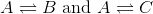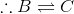# High School Physics : Understanding the Zeroth Law of Thermodynamics

## Example Questions

### Example Question #1 : Thermodynamics

Three substances are added to a mug to make coffee: the coffee, which is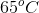, the milk, which is, and the sugar, which is in thermal equilibrium with the coffee. Describe the thermal state of the sugar.

The sugar is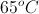, based on the second law of thermodynamics

The sugar is in equilibrium with the milk, based on the zeroth law of thermodynamics

Heat will be transferred from the coffee to the sugar to reach equilibrium

None of these conclusions are valid

Heat will be transferred from the sugar to the coffee to reach equilibrium

The sugar is in equilibrium with the milk, based on the zeroth law of thermodynamics

Explanation:

The zeroth law of thermodynamics states that if two objects are in thermodynamic equilibrium with a third object, then they must be in thermodynamic equilibrium with each other. In this question, the coffee is in equilibrium with both the milk and the sugar, allowing us to conclude that the milk and sugar must be in equilibrium with each other.

The second law of thermodynamics states that the entropy of the universe is always increasing, and is not relevant to this particular scenario.

### Example Question #2 : Thermodynamics

System A is in equilibrium with system C.

System B is in equilibrium with system C.

System A is in equilibrium with system B according to which law of thermodynamics?

The zeroth law of thermodynamics

The first law of thermodynamics

The second law of thermodynamics

The universal law of thermodynamics

The third law of thermodynamics

The zeroth law of thermodynamics

Explanation:

The zeroth law of thermodynamics states that if two separate systems are in equilibrium with a third system, then they are in equilibrium with each other. The zeroth law of thermodynamics is essentially equivalent to the transitive property of mathematics.

If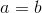and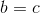, then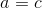.

The first law of thermodynamics states that internal energy changes due to heat flow. Mathematically, this law is presented as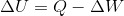.

The second law of thermodynamics states that the entropy (or disorder) of the universe is always increasing. Certain systems exist in which there is a local decrease in entropy, but these processes are always balanced by an increase of entropy outside of the system.

The third law of thermodynamics states that absolute zero is the state in which a system has zero entropy. Essentially, this means that it is impossible to reach absolute zero (at least with modern technology).

### Example Question #1 : Understanding The Zeroth Law Of Thermodynamics

Gas A is in thermal equilibrium with gases B and C. Which of the following is a valid conclusion?

Thermal equilibrium of gas B is directly proportional to that of gas C

Gases A and B have equal amounts of entropy

Thermal equilibrium of gas B is indirectly proportional to that of gas C

No conclusion can be drawn

Gases B and C are in thermal equilibrium with each other